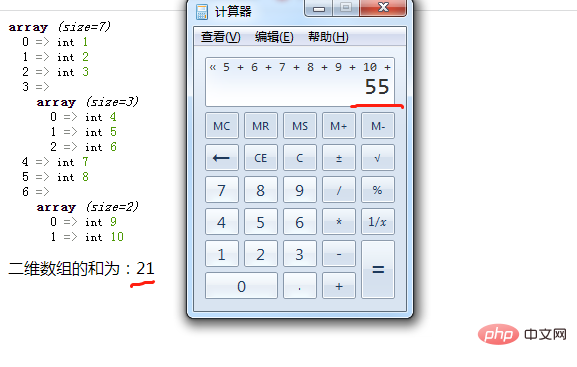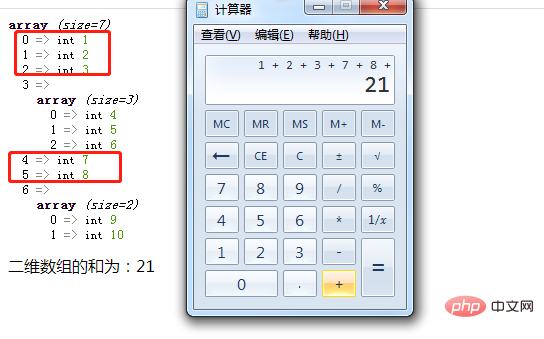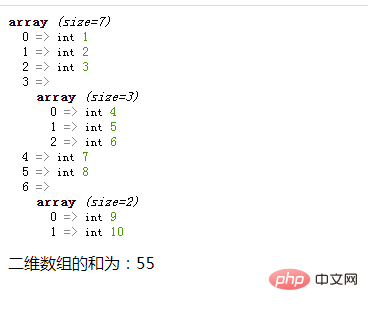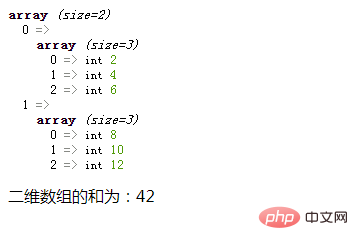# php二维数组求和用array_sum吗

php中二维数组求和只用array_sum()是不行的，因为array_sum()函数只处理一维数组；想要使用array_sum()实现二维数组求和，还需要借助foreach语句，语法“\$sum=0;foreach(\$arr as \$v){if(is_array(\$v)){\$sum+=array_sum(\$v);}else{\$sum+=\$v;}}”。php入门到就业线上直播课：进入学习

php二维数组只用array_sum()是无法求和的。

```<?php
\$arr = array(1,2,3,array(4,5,6),7,8,array(9,10));
var_dump(\$arr);
\$sum=array_sum(\$arr);
echo "二维数组的和为：".\$sum;
?>``````foreach(\$arr as \$v){
//循环体代码
}```
• 遍历给定的 \$arr 数组，在每次循环中将当前外层数组的值赋给 \$v。

• 如果不是数组类型，则将不是的外层元素相加求和

• 如果是数组类型，则使用array_sum()函数计算该内层数组中所有元素的和，并与外层元素和相加在一起即可。

```if(is_array(\$v)){
\$sum+=array_sum(\$v);
}else{
\$sum+=\$v;
}```

```function f(\$arr){
\$sum=0;
foreach(\$arr as \$v){
if(is_array(\$v)){
\$sum+=array_sum(\$v);
}else{
\$sum+=\$v;
}
}
echo "二维数组的和为：".\$sum."<br>";
}```

```\$arr = array(1,2,3,array(4,5,6),7,8,array(9,10));
var_dump(\$arr);
f(\$arr);``````\$arr = array(array(2,4,6),array(8,10,12));
var_dump(\$arr);
f(\$arr);```#### 相关文章推荐

• ❤️‍🔥共22门课程，总价3725元，会员免费学• php数组合并保留全部元素可以吗• php数组的元素类型必须一样吗• php数组键名能省略吗• php数组传递是引用传值吗1/1